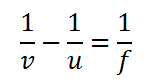# Thin Lens Equation

This CalcTown calculator calculates the image distance when the focal length and the object distance are given using Cartesian Coordinate Convention.

CARTESIAN CONVENTION:

1) Light initially propagates from left to right.

2) The origin of the Cartesian coordinate system is at the centre of the optical component.

3) Distances measured normal to the optic axis are positive above and negative below.

4) We denote object space distances as l, h, f, and image space distances as l ', h', f '.

5) Acute angles are positive when produced by anticlockwise rotation from the optic axis, and negative when produced by clockwise rotation.

cm
cm

#### Result

cmwhere

v=Image distance

u=Object distance

f=Focal length of the thin lens